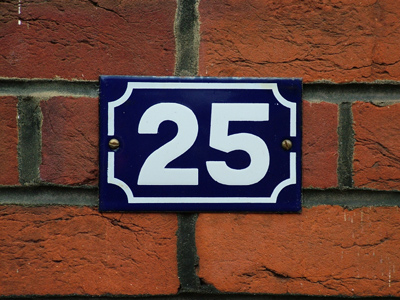The square number immediately after 16 is 25.

# Level 3-4 Algebra - Square Numbers

No doubt during your KS3 Maths lessons you've come across powers. You may even have done our quiz on the subject. Powers are the small numbers above and to the right of another number. Basically, they tell you to multiply that number by itself as many times as the power requires.

The power you will come across most often in algebra is the square. This is the most basic power and it means that you should multiply the number by itself just the once. So, if square numbers are the result of multiplying numbers by themselves, then you'll know quite a few already from your times tables. As an example 52 means 5 x 5 which everyone knows is 25.

See how much you remember from your lessons by playing the following quiz on square numbers. Read each question carefully and take your time. And don't forget the helpful comments after each question. They can help to clarify anything you are unsure of,

1.
How would you refer to 32?
Large 3, little 2
3, superscript 2
3 multiplied by 2
3 squared
You might say '3 to the power of 2' but it is more usual to say '3 squared'
2.
The roof of a rabbit hutch is completely tiled. There are 7 tiles across and 7 tiles down. How many tiles are there altogether?
14
28
42
49
Can you see why the number of tiles might be referred to as 72?
3.
What is the square number that comes immediately before 36?
10
15
20
25
6 x 6 is 36; 5 x 5 is 25
4.
What is the next square number after 9?
12
14
16
18
3 x 3 is 9; 4 x 4 is 16
5.
A patch of garden is 10 metres x 10 metres. What is its area expressed in square metres?
210
102
2 x 10
10 x 2
6.
What number is 32?
3
6
9
12
Multiply 3 by itself (3) and you get 9
7.
What is the missing square number in the sequence 1, 4, 9, 16, 25, 36, 49, ......., 81?
56
60
64
68
64 is 8 x 8; on one side it has 7 x 7 (49) and on the other side it has 9 x 9 (81)
8.
What is 02?
0
2
4
8
No matter how many times you multiply 0 x 0 it will still be 0!
9.
What is 12?
0
1
2
3
1 x 1 = 1
10.
Is the number 13,692 a square number?
Yes
No
Only in algebra
Always, except in algebra
A square number (also called a perfect square) is a positive whole number that is formed by multiplying a whole number with itself. For example, 16 = 4 x 4; 1 = 1 x 1; 1,681 = 41 x 41, and so on
You can find more about this topic by visiting BBC Bitesize - What are square and cube numbers

Author:  Frank Evans